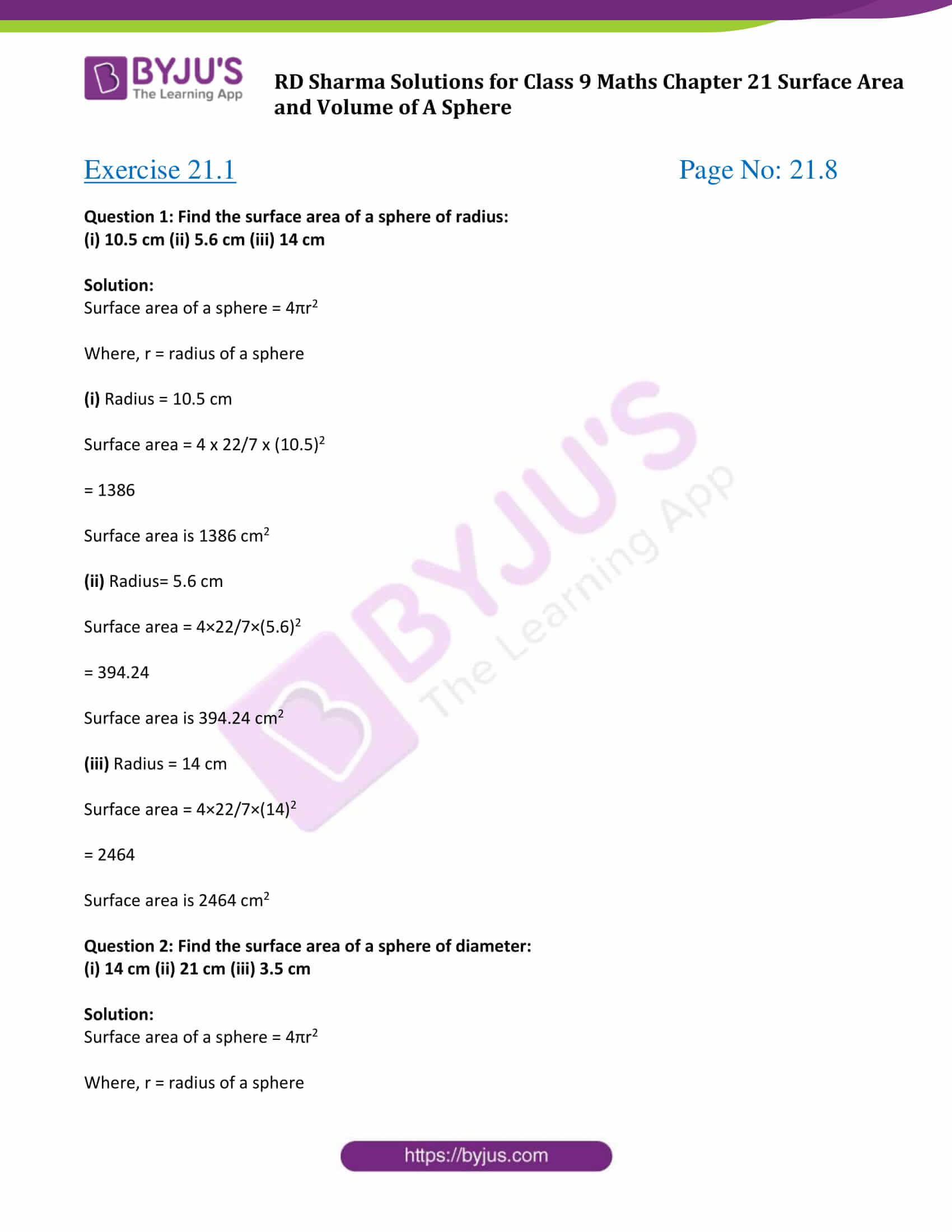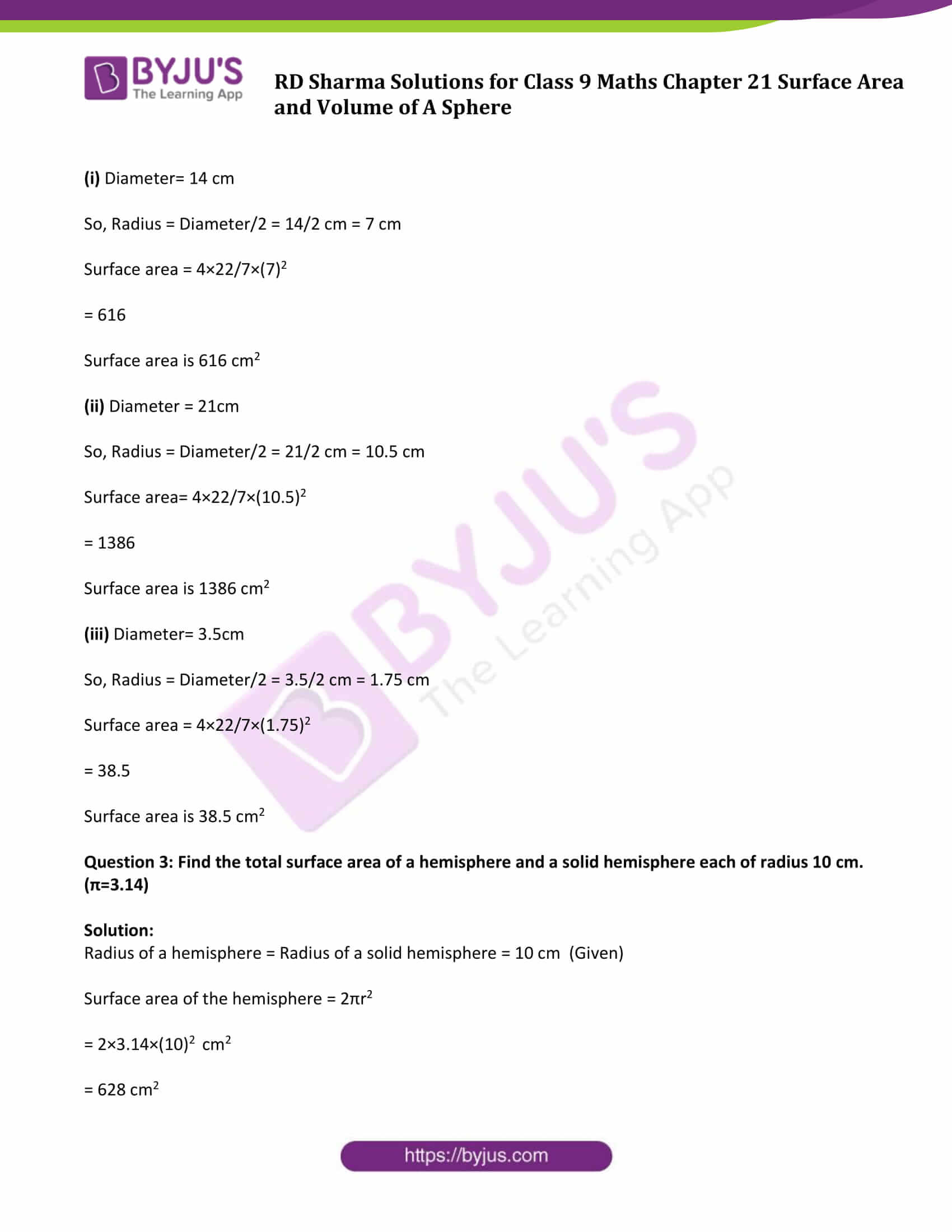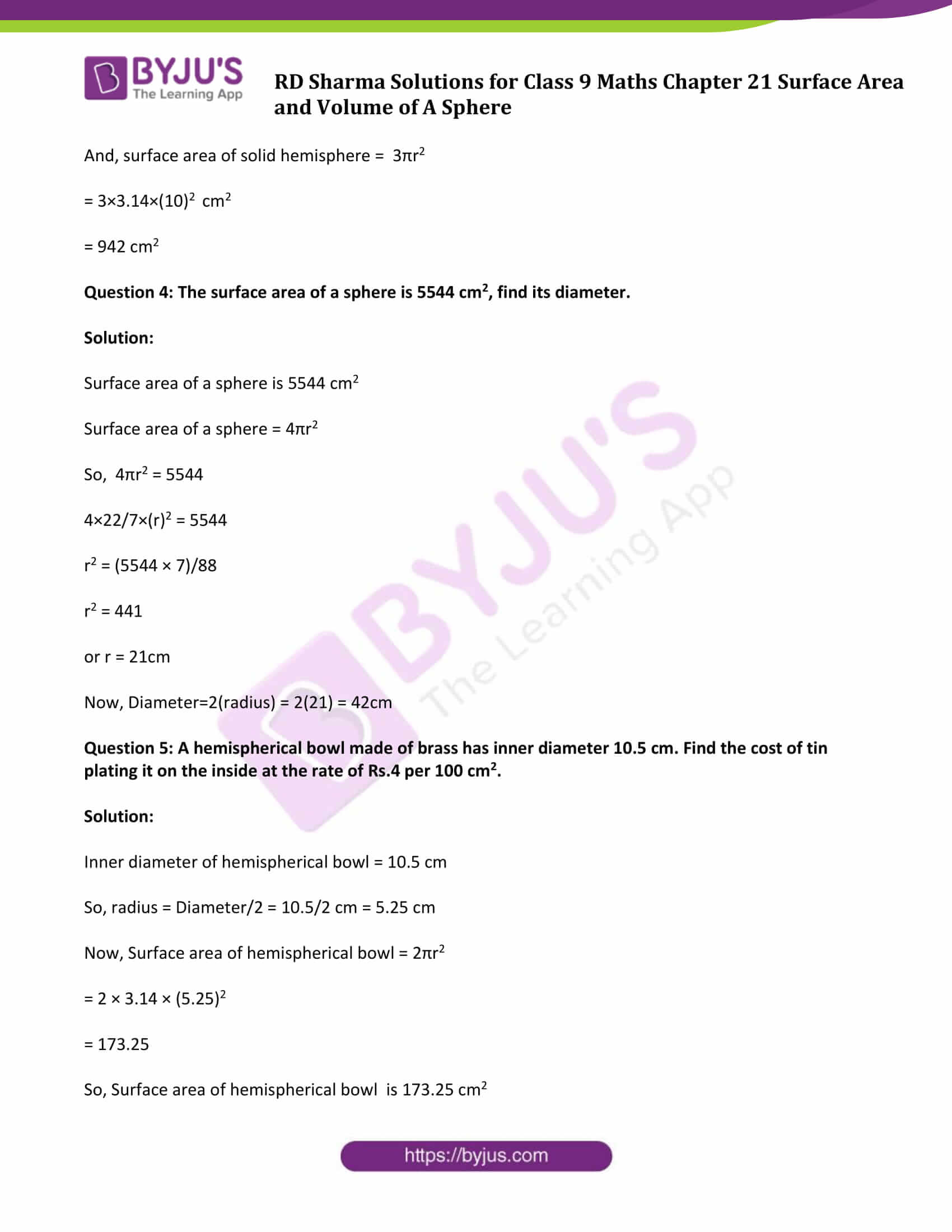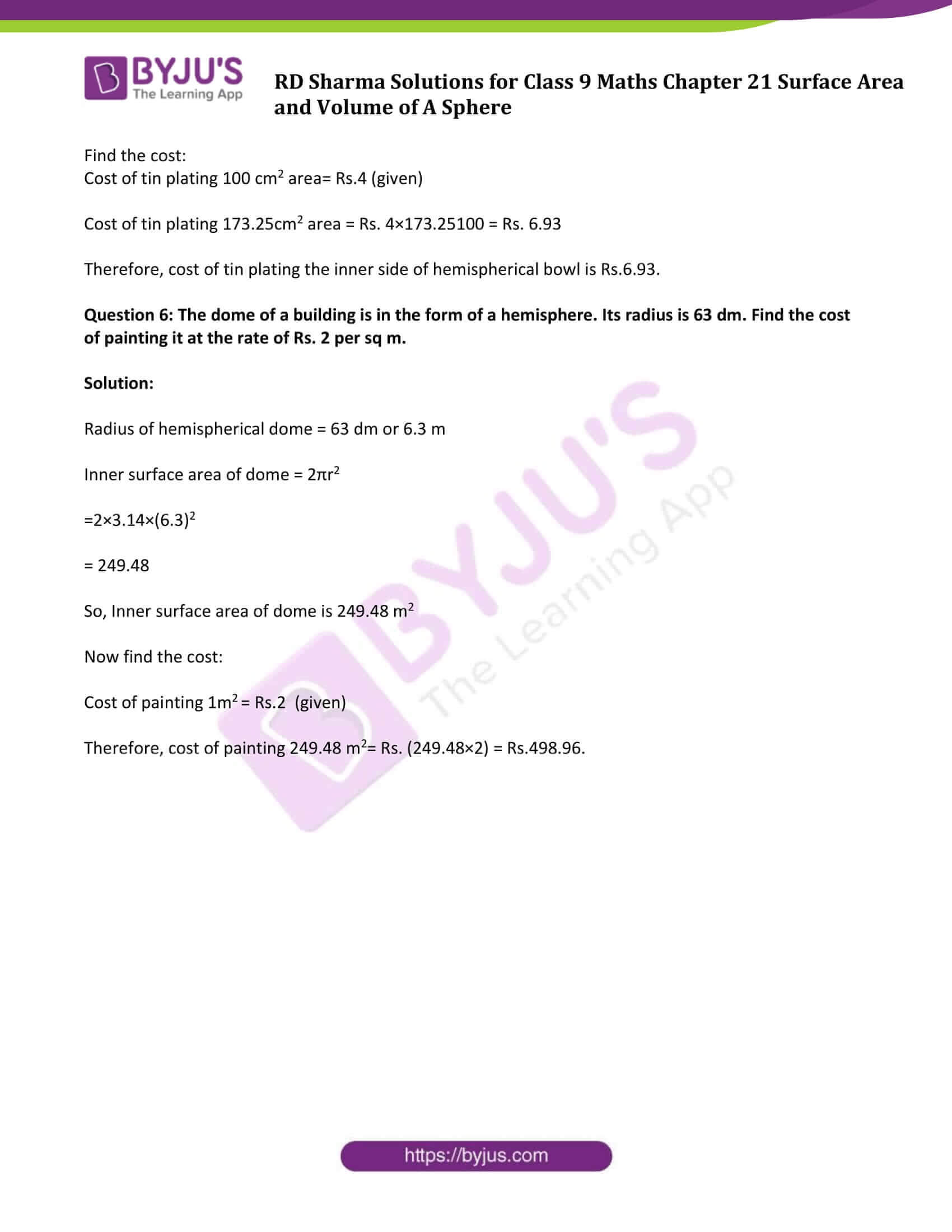# RD Sharma Solutions Class 9 Maths Chapter 21 Surface Area And Volume Of Sphere Exercise 21.1

Class 9 Chapter 21 – Surface Area and Volume of A Sphere Exercise 21.1 solutions are provided here. This study material is an invaluable aid to students when they need help with their homework while preparing for exams and while learning. Here all solutions to questions in RD Sharma textbook Solutions class 9 are given in a detailed and step by step format to help the students understand the concepts in an easy way. Click on the link below to get your pdf now.

## Download PDF of RD Sharma Solutions for Class 9 Maths Chapter 21 Surface Area and Volume of A Sphere Exercise 21.1### Access Answers to Maths RD Sharma Solutions for Class 9 Chapter 21 Surface Area and Volume of A Sphere Exercise 21.1 Page number 21.8

Question 1: Find the surface area of a sphere of radius:

(i) 10.5 cm (ii) 5.6 cm (iii) 14 cm

Solution:

Surface area of a sphere = 4πr2

Where, r = radius of a sphere

Surface area = 4 x 22/7 x (10.5)2

= 1386

Surface area is 1386 cm2

Surface area = 4×22/7×(5.6)2

= 394.24

Surface area is 394.24 cm2

Surface area = 4×22/7×(14)2

= 2464

Surface area is 2464 cm2

Question 2: Find the surface area of a sphere of diameter:

(i) 14 cm (ii) 21 cm (iii) 3.5 cm

Solution:

Surface area of a sphere = 4πr2

Where, r = radius of a sphere

(i) Diameter= 14 cm

So, Radius = Diameter/2 = 14/2 cm = 7 cm

Surface area = 4×22/7×(7)2

= 616

Surface area is 616 cm2

(ii) Diameter = 21cm

So, Radius = Diameter/2 = 21/2 cm = 10.5 cm

Surface area= 4×22/7×(10.5)2

= 1386

Surface area is 1386 cm2

(iii) Diameter= 3.5cm

So, Radius = Diameter/2 = 3.5/2 cm = 1.75 cm

Surface area = 4×22/7×(1.75)2

= 38.5

Surface area is 38.5 cm2

Question 3: Find the total surface area of a hemisphere and a solid hemisphere each of radius 10 cm. (π=3.14)

Solution:

Radius of a hemisphere = Radius of a solid hemisphere = 10 cm (Given)

Surface area of the hemisphere = 2πr2

= 2×3.14×(10)2 cm2

= 628 cm2

And, surface area of solid hemisphere = 3πr2

= 3×3.14×(10)2 cm2

= 942 cm2

Question 4: The surface area of a sphere is 5544 cm2, find its diameter.

Solution:

Surface area of a sphere is 5544 cm2

Surface area of a sphere = 4πr2

So, 4πr2 = 5544

4×22/7×(r)2 = 5544

r2 = (5544 × 7)/88

r2 = 441

or r = 21cm

Now, Diameter=2(radius) = 2(21) = 42cm

Question 5: A hemispherical bowl made of brass has inner diameter 10.5 cm. Find the cost of tin plating it on the inside at the rate of Rs.4 per 100 cm2.

Solution:

Inner diameter of hemispherical bowl = 10.5 cm

So, radius = Diameter/2 = 10.5/2 cm = 5.25 cm

Now, Surface area of hemispherical bowl = 2πr2

= 2 × 3.14 × (5.25)2

= 173.25

So, Surface area of hemispherical bowl is 173.25 cm2

Find the cost:

Cost of tin plating 100 cm2 area= Rs.4 (given)

Cost of tin plating 173.25cm2 area = Rs. 4×173.25100 = Rs. 6.93

Therefore, cost of tin plating the inner side of hemispherical bowl is Rs.6.93.

Question 6: The dome of a building is in the form of a hemisphere. Its radius is 63 dm. Find the cost of painting it at the rate of Rs. 2 per sq m.

Solution:

Radius of hemispherical dome = 63 dm or 6.3 m

Inner surface area of dome = 2πr2

=2×3.14×(6.3)2

= 249.48

So, Inner surface area of dome is 249.48 m2

Now find the cost:

Cost of painting 1m2 = Rs.2 (given)

Therefore, cost of painting 249.48 m2= Rs. (249.48×2) = Rs.498.96.

## RD Sharma Solutions for Class 9 Maths Chapter 21 Surface Area and Volume of A Sphere Exercise 21.1

RD Sharma Solutions Class 9 Maths Chapter 21 Surface Area and Volume of A Sphere Exercise 21.1 is based on following topics:

• Section of a sphere by a plane
• Hemisphere
• Spherical shell
• Surface Area of a sphere
• Surface Area of a hemisphere and
• Surface Area of a spherical shell